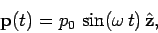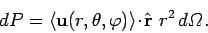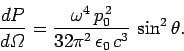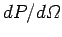Next: Thompson scattering Up: Electromagnetic radiation Previous: The Hertzian dipole

In the previous section, we examined the radiation emitted by a short electric dipole of oscillating dipole moment(1116)

where. We found that, in the far field, the mean electromagnetic energy flux takes the form [see Eq. (1094)](1117)

assuming that the dipole is centered on the origin of our spherical polar coordinate system. The mean power radiated into the element of solid angle, centered on the angular coordinates (,), is(1118)

Hence, the differential power radiated into this element of solid angle is simply(1119)

This formula completely specifies the radiation pattern of an oscillating electric dipole (provided that the dipole is much shorter in length than the wave-length of the emitted radiation). Of course, the power radiated into a given element of solid angle is independent of, otherwise energy would not be conserved. Finally, the total radiated power is the integral ofover all solid angles.Next: Thompson scattering Up: Electromagnetic radiation Previous: The Hertzian dipole
Richard Fitzpatrick 2006-02-02